Courses

# Retro (Past 13 Year) JEE Main (D Block Elements)

## 24 Questions MCQ Test Chemistry for IIT JEE (Class 12) | Retro (Past 13 Year) JEE Main (D Block Elements)

Description
This mock test of Retro (Past 13 Year) JEE Main (D Block Elements) for JEE helps you for every JEE entrance exam. This contains 24 Multiple Choice Questions for JEE Retro (Past 13 Year) JEE Main (D Block Elements) (mcq) to study with solutions a complete question bank. The solved questions answers in this Retro (Past 13 Year) JEE Main (D Block Elements) quiz give you a good mix of easy questions and tough questions. JEE students definitely take this Retro (Past 13 Year) JEE Main (D Block Elements) exercise for a better result in the exam. You can find other Retro (Past 13 Year) JEE Main (D Block Elements) extra questions, long questions & short questions for JEE on EduRev as well by searching above.
QUESTION: 1

### Which of the following arrangements does not represent the correct order of the property stated against it ? (JEE Main 2013)

Solution:

(a) V2+ = 3 unpaired electrons, Cr2+ = 4 unpaired electrons
Mn2+ = 5 unpaired electrons, Fe2+ = 4 unpaired electrons
Hence, the order of paramagnetic behaviour should be V2+ < Cr2+ < Fe2+ < Mn2+
(b) Ionic size decreases from left to right in the same period.
(c) (As per data from NCEFIT)
Co3+/Co2+ = 1.97; Fe3+/Fe2+ = 0.77;Cr3+/Cr2+ =-0.41, Sc3+ is highly stable (it does not show + 2).
(d) The oxidation state increases as we go from group 3 to group 7 in the same period.

QUESTION: 2

### Four successive members of the first row transition elements listed below with atomic numbers. Which one of them is expected to have the highest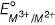value ? (JEE Main 2013)

Solution:

SRP value normally increases from left to right in the period of cf-block elements. Some SRP value are exceptionally higher due to stability of product ion, e.g.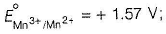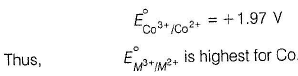QUESTION: 3

### Iron exhibits +2 and +3 oxidation states. Which of the following statements about iron is incorrect? (AIEEE 2012)

Solution:

(a) FeO > Fe2O3 (basic). Thus, it is correct statement.
(b) FeCI2 > FeCI3 (ionic), larger the charge, greater the polarising power. Thus, greater the covalent nature. Thus, the given statement is correct.
(c) Fe2+ salts are more ionic thus less volatile than Fe3+ salts. Thus, given statement is correct.
(d) Greater the covalent nature, more easily they are hydrolysed, Thus, FeCI3 is more hydrolysed than FeCI2. Thus, statement (d) is incorrect.

QUESTION: 4

In context of the lanthanides, which of the following statements is not correct?

(AIEEE 2011)

Solution:

(a) There is a gradual decrease in the radii of the lanthanoids with increasing atomic number a case of lanthanide contraction, thus true.
(b) Ionisation potential for the formation of Lu3+ is comparatively low, hence +3 state is favourable, thus true,
(c) Due to lanthanoid contraction, Zr and Hf; Nb and Ta,
Mo and W have the same size and thus, similar properties and thus separation is notfwsy, thus true.
(d) Formation of +4 state requires very high energy, thus incorrect.

QUESTION: 5

The outer electronic configuration of Gd (Atomic number 64) is

(AIEEE 2011)

Solution: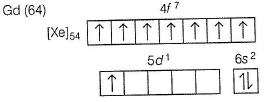All the electrons in 47-orbital are unpaired, hence stable. Thus,
Gd (64) has EC as [Xe]54 4f7, 5d\ 6s2 instead of [Xe]54 4f8, 6s2.

QUESTION: 6

The correct order of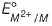values with negative sign for the four successive elements Cr, Mn, Fe and Co is

(AIEEE 2010)

Solution:

Usually across the first transition series, the negative values for standard electrode potential decrease except for Mn due to stabled5-configuration. So, correct order: Mn > Cr > Fe > Co

QUESTION: 7

Knowing that the chemistry of lanthanides (Ln) is dominated by its +3 oxidation state, which of the following statements is incorrect?

Solution:

The most common oxidation state of lanthanoid is +3. Lanthanoids in +3 oxidation state usually have unpaired electron in f-subshell and impart characteristic colour in solid as well as in solution state due to f-f transition.

(Except lanthanum and lutetium)

QUESTION: 8

In context with the transition elements, which of the following statements is incorrect?

(AIEEE 2009)

Solution:

As oxidation state increases, electronegativity increases thus acidic character increases not basic.

QUESTION: 9

Larger number of oxidation states are exhibited by the actinides than those by the lanthanides, the main reason being

(AIEEE 2008)

Solution:

Lanthanoids : [Xe] f1-145d0-16s2
Actinoids : [Rn]5f1-14 6d0-17s2
Lanthanoids and actinoids use core d and f-orbitals also to show higher oxidation state. As actinoids have comparatively low energy difference between f and d-orbitals, thus they show more number of oxidation states.

QUESTION: 10

Identify the incorrect statement among the following.

(AIEEE 2007)

Solution:

4f-orbital is nearer to nucleus as compared to 5f-orbital. Therefore, shielding of 4f is more than 5f-orbital.

QUESTION: 11

The actinides exhibit more number of oxidation states in general than the lanthanides. This is because

(AIEEE 2007)

Solution:

The actinoids (5f-element) exhibit more number of oxidation states in general than the lanthanoids because 5f-orbitals extend farther from the nucleus than the 4f-orbitals.
Thus, the attraction on the 5f-electrons in actinoid series decreases. Hence, they can easily participate in bonding and therefore, exhibits more number of oxidation states.

QUESTION: 12

Lanthanides contraction is caused due to

(AIEEE 2006)

Solution:

Imperfect shielding on outer electrons by 4f-electrons from the nuclear charge is due to the diffused large shape of 4f-orbitals. This imperfect shielding is unable to counterbalance the effect of the increased nuclear charge. Hence, the net result shows contraction in size.

QUESTION: 13

On heating, mixture of Cu2O and CU2S will give

(AIEEE 2005)

Solution:

Following reaction takes place during Bessemerisation
2Cu2O + CU2S → 6Cu + SO2

QUESTION: 14

Calomel (Hg2CI2)on reaction with ammonium hydroxide gives

(AIEEE 2005)

Solution: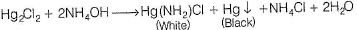QUESTION: 15

Cerium (Z = 58) is an important member of the lanthanides. Which of the following statements about cerium is incorrect?

(AIEEE 2004)

Solution:

+3 and +4 states are shown by Ce in aqueous solution. Thus, statement (c) is incorrect.

QUESTION: 16

Of the following outer electronic configurations of atoms, the highest oxidation state is achieved by which one of them ?

(AIEEE 2004)

Solution: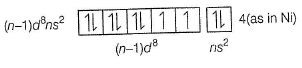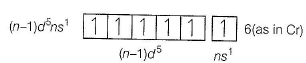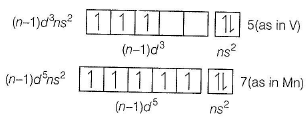QUESTION: 17

Which of the following groups of transition metals is called coinage metals?

(AIEEE 2003)

Solution:

Cu, Ag, Au are called coinage metals because in the early times, they were used in making coins.

QUESTION: 18

Which one of the following nitrates will leave behind a metal on strong heating?

(AIEEE 2003)

Solution:

Metal oxides lying below Hg in electrochemical series decompose to form metal.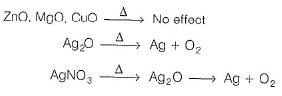QUESTION: 19

For making good quality mirrors, plates of float glass are used. These are obtained by floating molten glass over a liquid metal which does not solidify before glass. The metal used can be

(AIEEE 2003)

Solution:

Mercury (Hg) solidifies after glass, so used mercury for making good quality mirrors.

QUESTION: 20

Which one of the following statements is correct?

(AIEEE 2003)

Solution:

Ammonia can dissolve precipitate of AgCI due to the formation of complex as given below.
AgCI + 2NH3 → [Ag(NH3)2CI]

QUESTION: 21

A red solid is insoluble in water. However, it becomes soluble if some Kl is added to water. Heating the red solid in a test tube results in liberation of some violet coloured fumes and droplets of a metal appear on the cooler parts of the test tube. The red solid is

(AIEEE 2003)

Solution:

Hgl2 becomes soluble in water, when Kl is added to it due to the formation of K2Hgl4 complex which is soluble in water.
Hgl2 + 2KI → K2Hgl4(Soluble)
But when Hgl2 is heated, Hgl2 decomposes to give violet coloured fumes of I2 that deposits on the cooler parts of the test tube.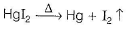QUESTION: 22

What would happen when a solution of potassium chromate is treated with an excess of dilute nitric acid?

(AIEEE 2003)

Solution: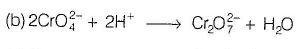QUESTION: 23

Most common oxidation states of Ce (cerium) are

(AIEEE 2002)

Solution:

Cerium, Ce58 = [Xe] 4f15d16s2. Its most stable oxidation state is +3 but +4 is also existing.

QUESTION: 24

Which of the following ions has the maximum magnetic moment?

(AIEEE 2002)

Solution:

Larger the number of unpaired electrons, greater the magnetic moment,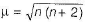where, n = Number of unpaired electrons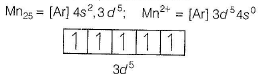Number of unpaired electrons = 5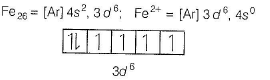Number of unpaired electrons = 4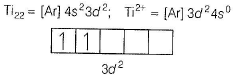Number of unpaired electrons = 2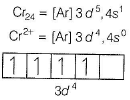Number of unpaired electrons = 4
Hence, Mn2+: [Ar] 3d5 with five unpaired electrons have maximum magnetic moment.